# Leetcode 40组合总数(回溯)Ⅱ&41缺失的第一个正数&42接雨水

## 组合总数(回溯)

andidates 中的每个数字在每个组合中只能使用一次。

示例 1:

[
[1, 7],
[1, 2, 5],
[2, 6],
[1, 1, 6]
]

[
[1,2,2],

]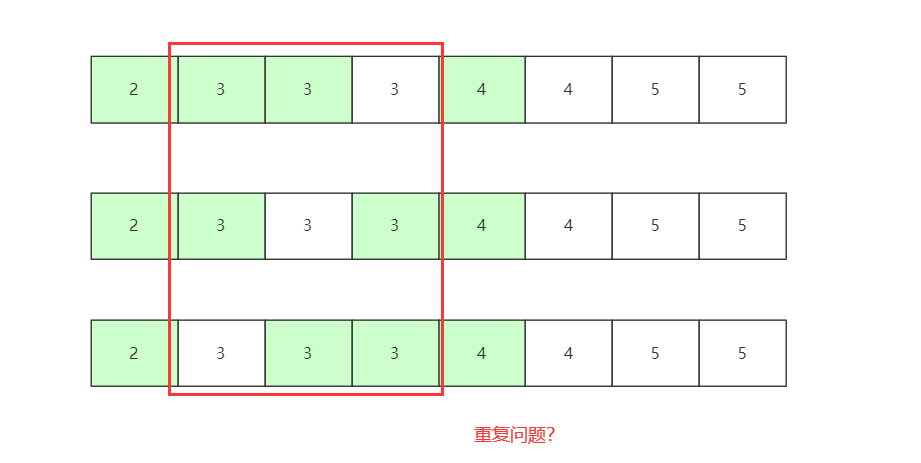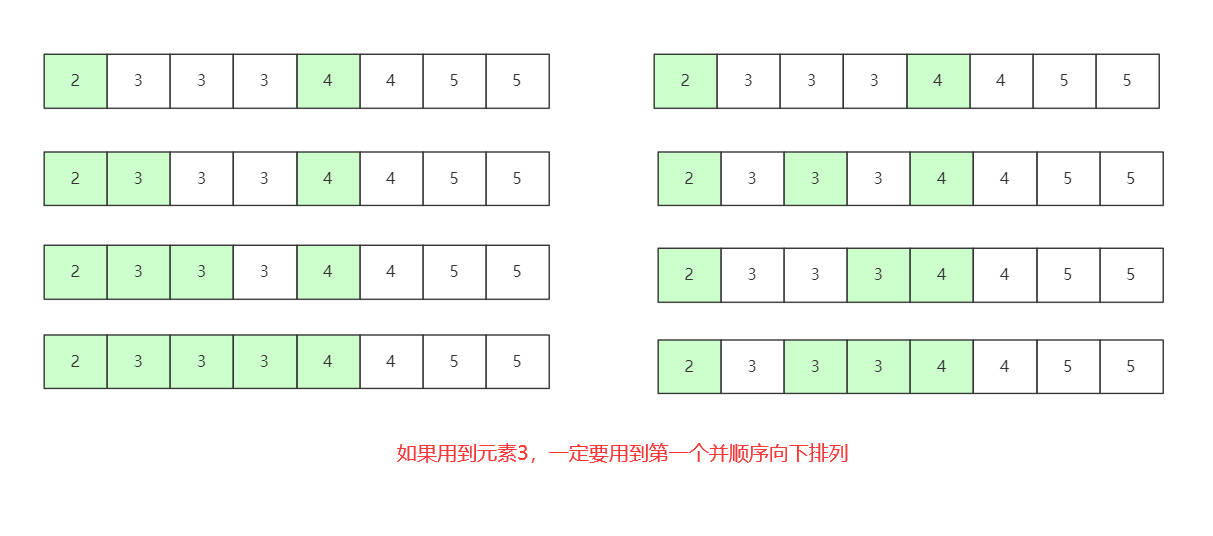List<Integer> list = new ArrayList<Integer>();// list用来回溯过程中储存元素
List<List<Integer>> val = new ArrayList<List<Integer>>();// 结果

public List<List<Integer>> combinationSum2(int[] candidates, int target) {
Arrays.sort(candidates);
dfs(0, candidates, target);
return val;
}

/*
* index 表示当前编号 count 表示当前数值的和
*/
private void dfs(int index, int[] candidates, int target) {
// System.out.println(list.toString());
if (target == 0) {
return;
} else if (index == candidates.length) {
return;
} else {
int r = index;
while (r < candidates.length && candidates[index] == candidates[r]) {
r++;
}
int i;
for (i = index; i < r; i++) {
target -= candidates[i];
if (target>=0) {
dfs(r, candidates, target);

} else {//没有执行dfs但是数值需要恢复 因为i还是变了
target += candidates[index];
list.remove(list.size() - 1);
break;
}
}
for (; i > index; i--) {
target += candidates[index];
list.remove(list.size() - 1);
}
dfs(r,  candidates, target);// 不使用该元素的
}
}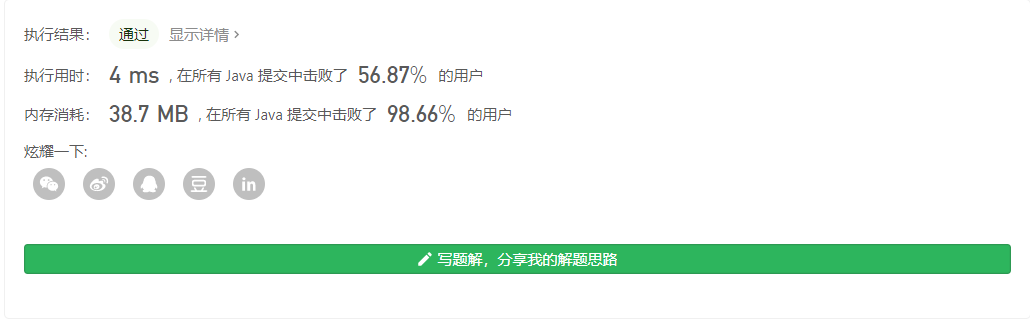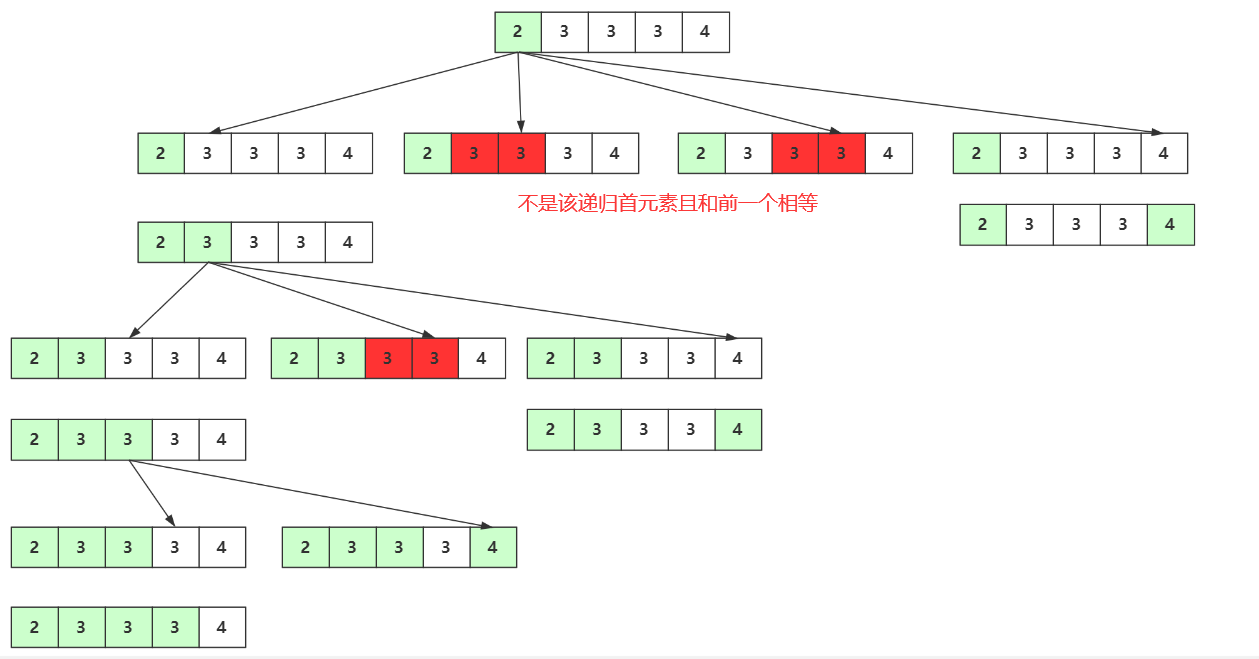List<Integer> list = new ArrayList<Integer>();// list用来回溯过程中储存元素
List<List<Integer>> val = new ArrayList<List<Integer>>();// 结果

public List<List<Integer>> combinationSum2(int[] candidates, int target) {
Arrays.sort(candidates);
dfs(0, candidates, target);
return val;
}

/*
* index 表示当前编号 count 表示当前数值的和
*/
private void dfs(int index, int[] candidates, int target) {

if (target == 0) {
return;
}
for(int i=index;i<candidates.length;i++)
{
if(target-candidates[i]<0)break;
if(i!=index&&candidates[i]==candidates[i-1])continue;
dfs(i+1, candidates, target-candidates[i]);
list.remove(list.size()-1);
}
}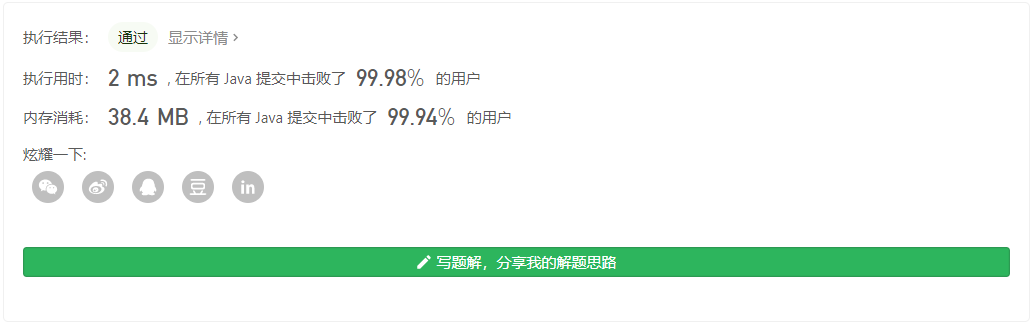## 缺失的第一个正数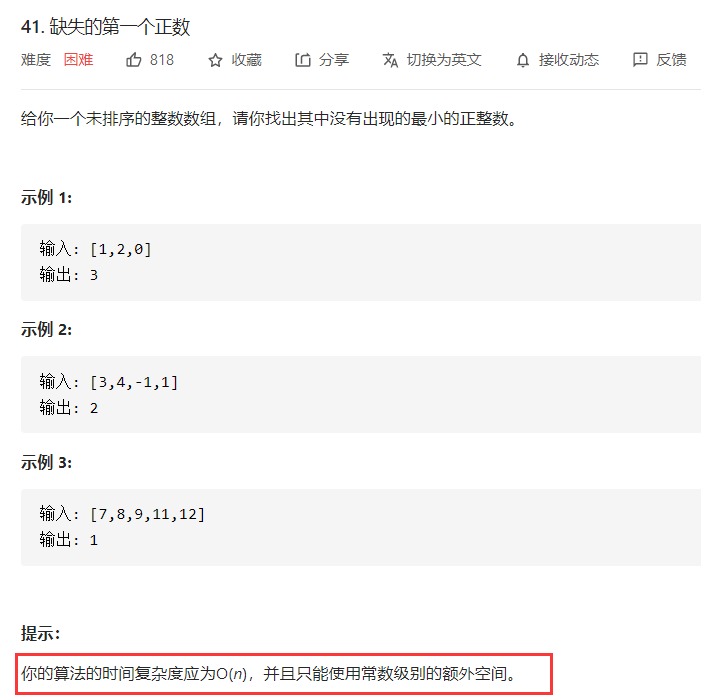public int firstMissingPositive(int[] nums) {
int time[]=new int[nums.length+1];
for(int i=0;i<nums.length;i++)
{
if(nums[i]>0&&nums[i]<nums.length)
{
time[i]++;
}

}
for(int i=1;i<time.length;i++)
{
if(time[i]==0)
return i;
}
return nums.length;
}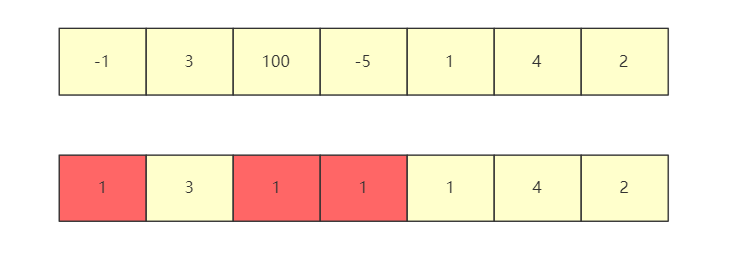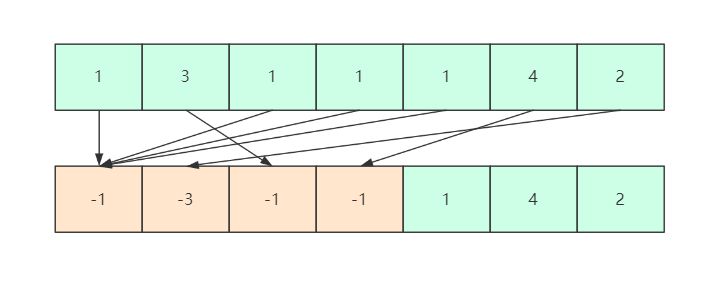public int firstMissingPositive(int[] nums) {
boolean jud =false;
//第一轮循环，将无用的数字置为1 如果整个数列中没有1 那么将返回1
for(int i=0;i<nums.length;i++)
{
if(nums[i]<=1||nums[i]>nums.length)
{
if(nums[i]==1)jud=true;
nums[i]=1;
}
}
if(!jud)return 1;
//第二轮循环，所有数字现在目前的状态是1 - nums.length区间内
for(int i=0;i<nums.length;i++)
{
int index=Math.abs(nums[i])-1;//该位置对应的索引

if(nums[index]>0) {nums[index]=-nums[index];}
}
for(int i=0;i<nums.length;i++)
{
if(nums[i]>0)return i+1;
}
return nums.length+1;
}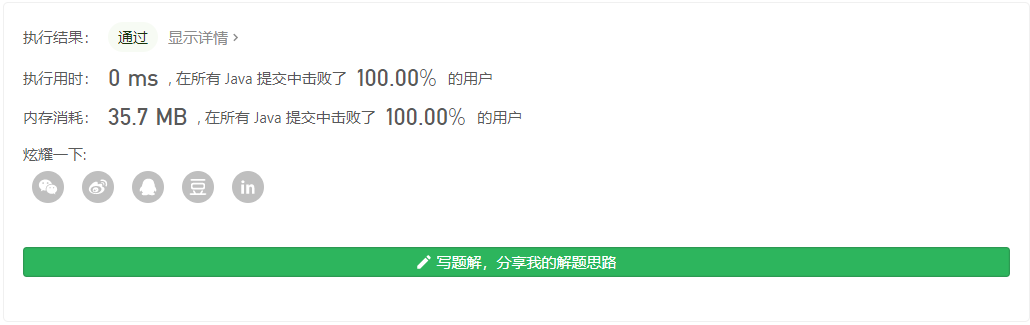## 接雨水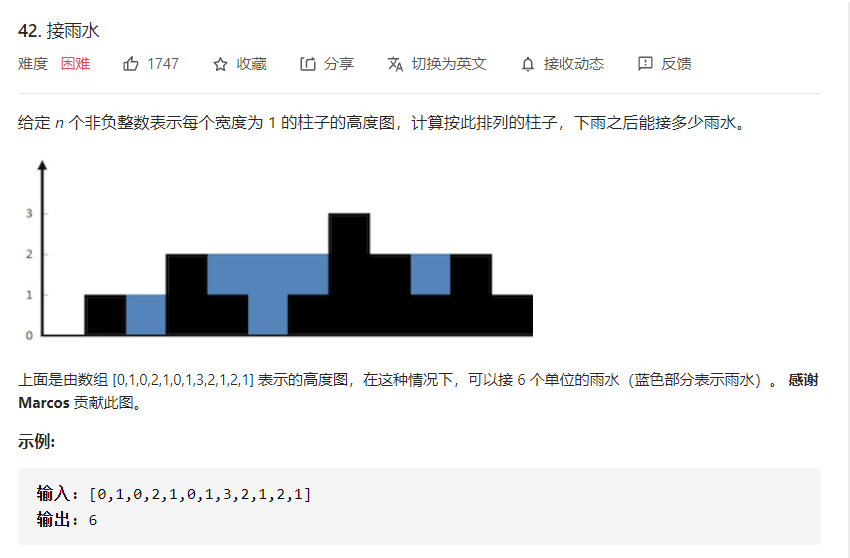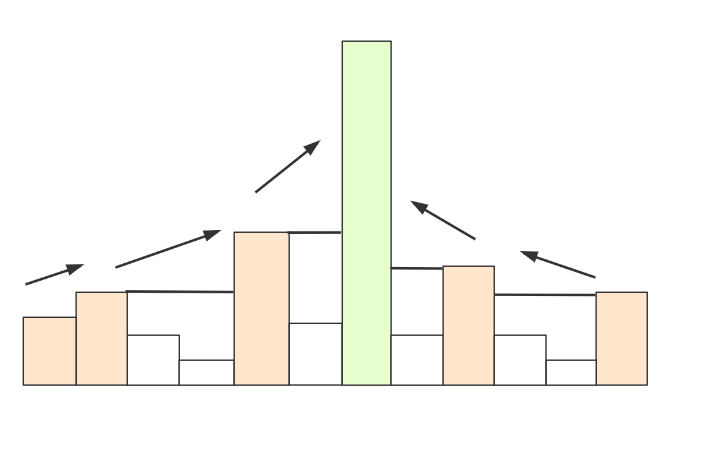• 左右进行相等操作，从左来看，需要一个llteam,让lteam往右试探.
• 试探途中如果height[lteam]<height[l],继续前进，后面一定会有更大的。
• 试探途中如果height[lteam]>height[l],计算途中雨点和，然后l赋值为lteam，继续试探。

public int trap(int[] height) {
int l=0,r=l+1;
int count=0;
int max=0,maxindex=0;
while (l<height.length&&r<height.length) {
if(height[r]>=height[l])//找到更大的靠山
{
for(int i=l;i<r;i++)
{
count+=height[l]-height[i];
}
l=r;
r++;
max=0;maxindex=0;
}
else {//不大于
if(height[r]>=max)
{
max=height[r];
maxindex=r;
}

r++;
if(r==height.length)
{
for(int i=l+1;i<maxindex;i++)
{
count+=max-height[i];
}
l=maxindex;r=l+1;
max=0;maxindex=0;
}
}
}
return count;
}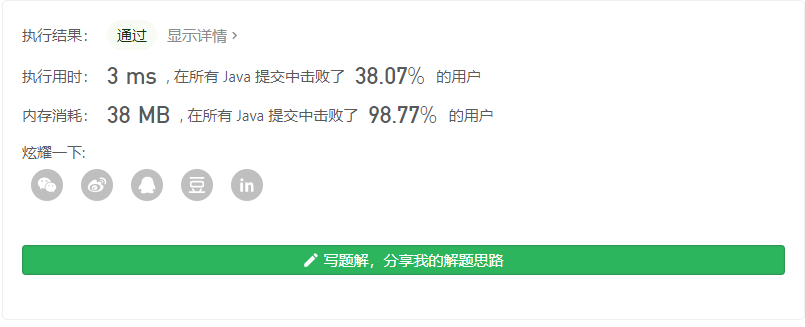public int trap(int[] height) {
int l=0,r=l+1;
int count=0;

while (l<height.length&&r<height.length) {
if(height[r]>=height[l])//找到更大的靠山
{
for(int i=l;i<r;i++)
{
count+=height[l]-height[i];
}
l=r;r=l+1;
}
else {//不大于
r++;
if(r==height.length)//说明当前的left已经是天底下最大的值了
{
int max=0;
for(int i=r-1;i>l;i--)
{
if(height[i]>max)
{
max=height[i];
}
else
{
count+=max-height[i];
}
}
l=r;
}
}
}
return count;# 结语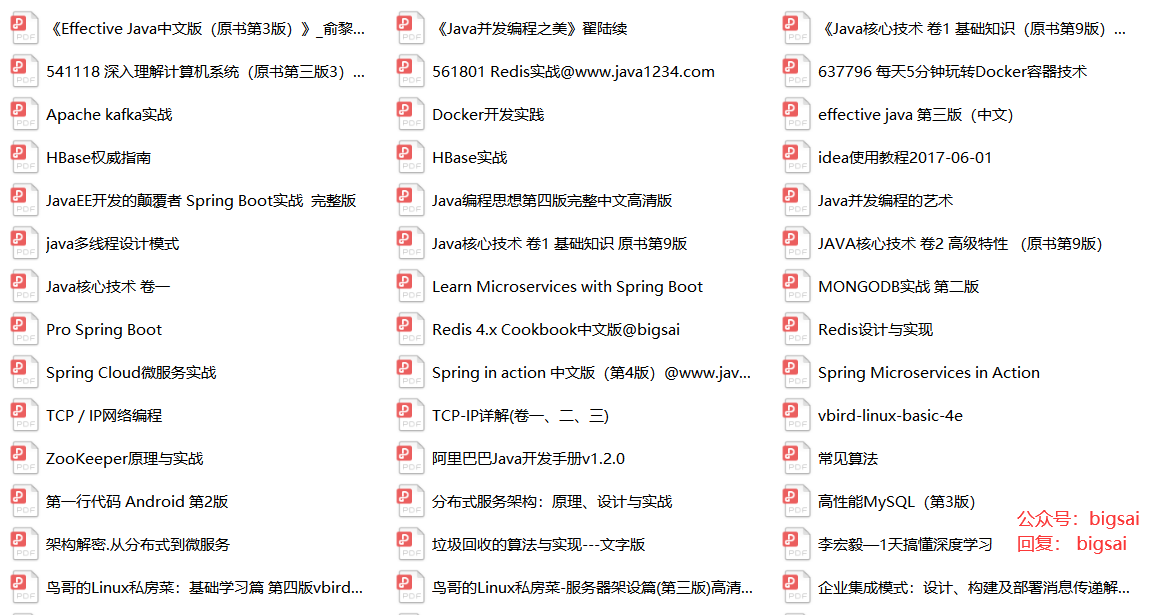Big sai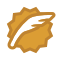CSDN认证博客专家 scikit-learn

11-076111-271803
08-25390
02-191万+
09-0284
02-05155
11-04102
08-053357